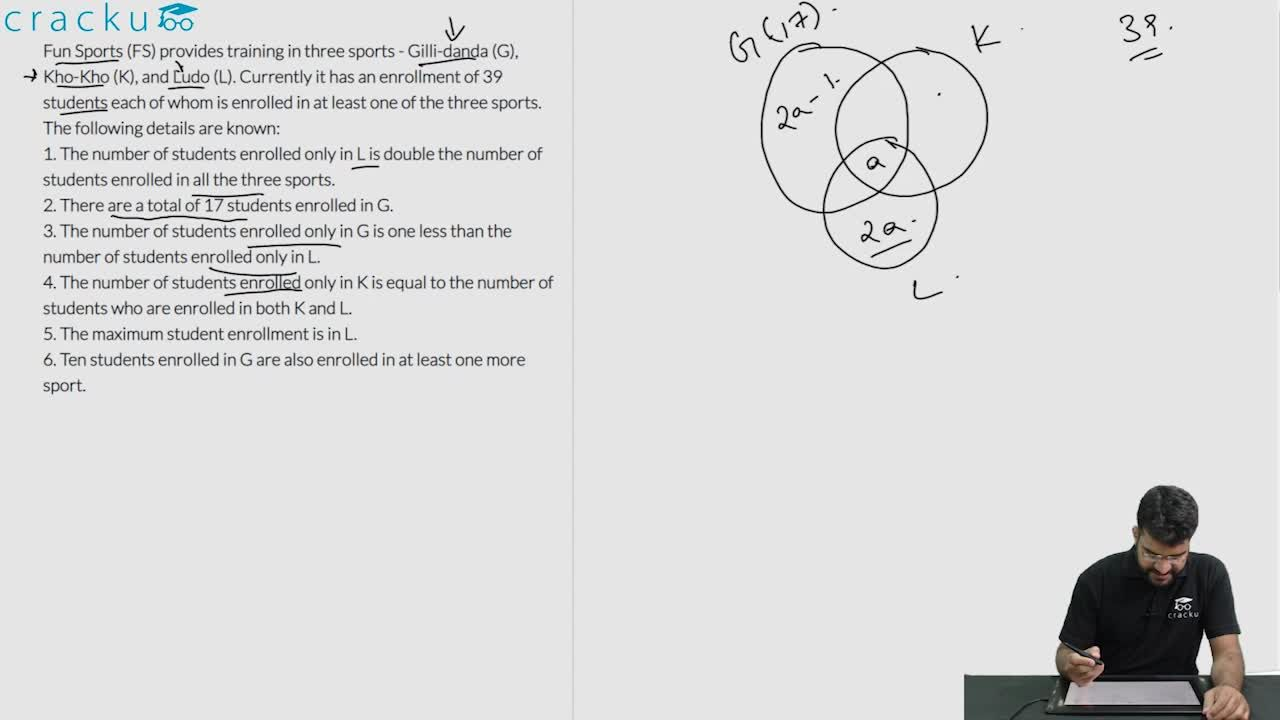### CAT 2018 Question Paper (Slot 2) - LRDI Question 15

Instructions

Fun Sports (FS) provides training in three sports - Gilli-danda (G), Kho-Kho (K), and Ludo (L). Currently it has an enrollment of 39 students each of whom is enrolled in at least one of the three sports. The following details are known:
1. The number of students enrolled only in L is double the number of students enrolled in all the three sports.
2. There are a total of 17 students enrolled in G.
3. The number of students enrolled only in G is one less than the number of students enrolled only in L.
4. The number of students enrolled only in K is equal to the number of students who are enrolled in both K and L.
5. The maximum student enrollment is in L.
6. Ten students enrolled in G are also enrolled in at least one more sport.

Question 15

# Due to academic pressure, students who were enrolled in all three sports were asked to withdraw from one of the three sports. After the withdrawal, the number of students enrolled in G was six less than the number of students enrolled in L, while the number of students enrolled in K went down by one. After the withdrawal, how many students were enrolled in both G and K?

Solution

Let 'x' be the number of students enrolled in all three sports. Then the number of students enrolled only in L = 2x

It is given that there are a total of 17 students enrolled in G. Also, ten students enrolled in G are also enrolled in at least one more sport. Hence, the number of students enrolled in only G = 17 - 10 = 7

The number of students enrolled only in G is one less than the number of students enrolled only in L. Hence, the number of students enrolled only in L = 7+1

$$\Rightarrow$$ 2x = 8

$$\Rightarrow$$ x = 4

Let us assume that 'y' students are enrolled in K and L but not G. Then, the number of students enrolled only in K = y + 4

Let us assume that 'z' be the the number of students enrolled in G and K but not L. Then, the number of students enrolled G and L bot not K = 10 - 4 - z = 6 - z

It is given that a total of 39 students in the sports.

7 + z + 4 + 6 - z + 8 + y + y + 4 = 39

$$\Rightarrow$$ y = 5

Number of students enrolled in G = 17

Number of students enrolled in K = 9 + 4 + 5 + z = 18 + z

Number of students enrolled in L = 6 - z + 4 + 5 + 8 = 23 - z

It is given that the maximum student enrollment is in L.

$$\Rightarrow$$ 23 - z > 18 + z

$$\Rightarrow$$ 2z < 5

$$\Rightarrow$$ z < 2.5

Therefore, we can say that z can take three values = {0, 1, 2}

Hence, the number of students enrolled in K = 18 + z = {18, 19, 20}

It is given that after withdrawal the number of students enrolled in K went down by one. This one student must have left sports K. Hence we can say that the remaining 3 students must have left either G or L.

Before withdraw there were a total of 24 students were enrolled in exactly 1 sports, 11 students were enrolled in exactly 2 courses and 4 students were enrolled in all three courses.

The students which were enrolled in all three sports, withdrew from one of the sports. Hence, we can say that now the number of students who were enrolled in exactly 2 courses = 11 + 4 = 15.

It is given that the number of students enrolled in G was six less than the number of students enrolled in L. Let 'a' be the number of students who were enrolled in G and K but not L. Then, the number of students who were enrolled in L and K but not G = a + 5

Consequently, we can say that the number of students enrolled in G and L but not K = 15 - (2a + 5) = 10 - 2a

Number of students enrolled in this case = a + a+5 + 9 = 14 + 2a. We can see that '14+2a' is an even number. It is given that the number of students enrolled in K went down by one. Therefore, we can say that the number of students enrolled in K earlier was an odd number.

Hence, the number of students enrolled in K = 18 + z = {18, 19, 20}

We can see that only '19' is an odd number. Hence, we can say that the number of students enrolled in K after withdrawal = 18

$$\Rightarrow$$ 14 + 2a = 18

$$\Rightarrow$$ a = 2

From the diagram we can see that the number of students enrolled in both G and K = 2.

### View Video Solution